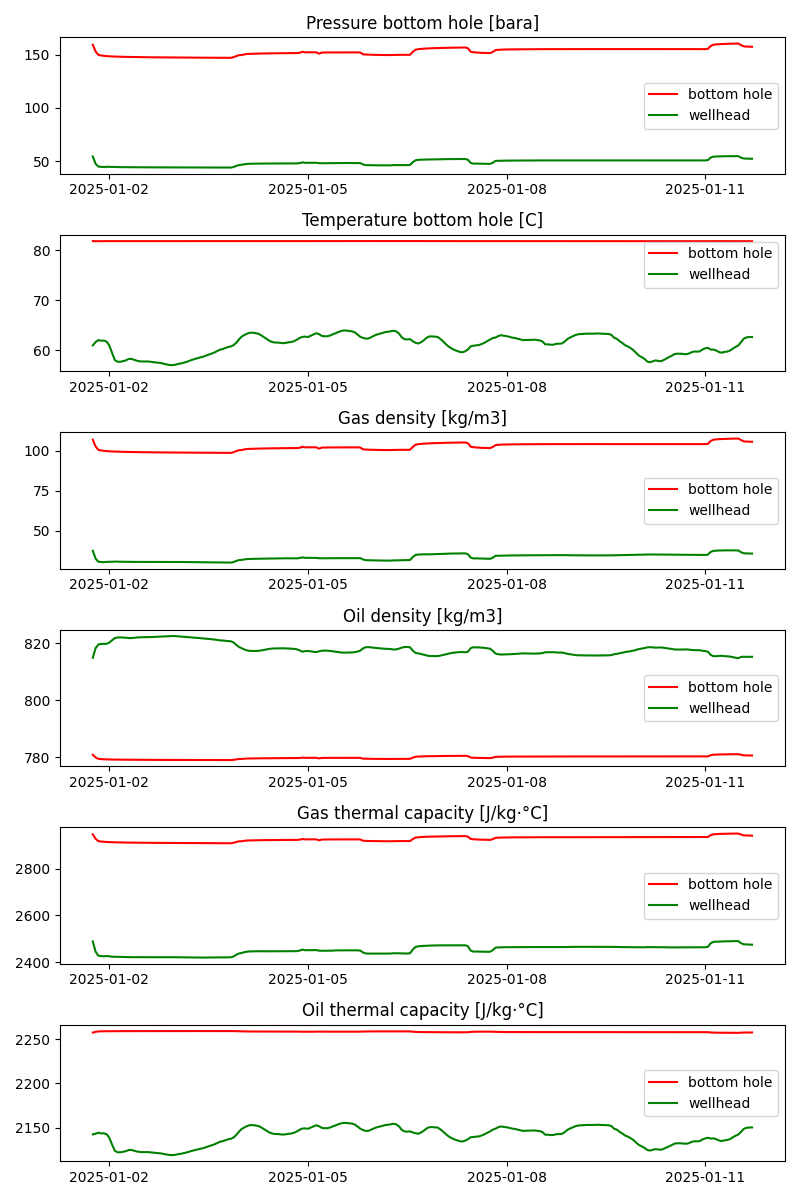# Calculate fluid properties given pressure and temperature

The calculation of fluid properties is a fundamental component of mass balance and other methods of conservation. The goal of this feature is to calculate the fluid properties given the pressure and temperature conditions and the composition of the fluid itself. Equation of state simulators output a fluid file that is a table of the fluid properties for a range of pressure and temperature conditions. The input to these simulators is the composition of the fluid obtained from lab tests. For this specific feature, the input fluid file is a .tab file used by OLGA, a transient multiphase flow simulator.```import os
import pickle as pkl

import matplotlib.dates as mdates
import matplotlib.pyplot as plt

from indsl.oil_and_gas.live_fluid_properties import retrieve_fluid_properties

base_path = "" if __name__ == "__main__" else os.path.dirname(__file__)

pbh = df.iloc[:, 0] * 100000
tbh = df.iloc[:, 1]
pwf = df.iloc[:, 2] * 100000
twf = df.iloc[:, 3]

pbh.name = "Pressure bottom hole [bara]"
tbh.name = "Temperature bottom hole [C]"

gas_den_bh = [retrieve_fluid_properties(pbh, tbh, pvt_data, param="ROG"), "red"]
gas_den_wh = [retrieve_fluid_properties(pwf, twf, pvt_data, param="ROG"), "green"]
liq_den_bh = [retrieve_fluid_properties(pbh, tbh, pvt_data, param="ROHL"), "red"]
liq_den_wh = [retrieve_fluid_properties(pwf, twf, pvt_data, param="ROHL"), "green"]
hl_bh = [retrieve_fluid_properties(pbh, tbh, pvt_data, param="CPHL"), "red"]
hl_wh = [retrieve_fluid_properties(pwf, twf, pvt_data, param="CPHL"), "green"]
hg_bh = [retrieve_fluid_properties(pbh, tbh, pvt_data, param="CPG"), "red"]
hg_wh = [retrieve_fluid_properties(pwf, twf, pvt_data, param="CPG"), "green"]

pbh = df.iloc[:, 0]
pwf = df.iloc[:, 2]

pbh.name = "Pressure bottom hole [bara]"
pbh = [pbh, "red"]
tbh = [tbh, "red"]
pwf = [pwf, "green"]
twf = [twf, "green"]

data = [[pbh, pwf], [tbh, twf], [gas_den_bh, gas_den_wh], [liq_den_bh, liq_den_wh], [hg_bh, hg_wh], [hl_bh, hl_wh]]

fig, ax = plt.subplots(figsize=(8, 2 * len(data)), nrows=len(data), ncols=1)
ax = ax.ravel()
for idx, i in enumerate(data):
ax[idx].plot(i, color=i, label="bottom hole")